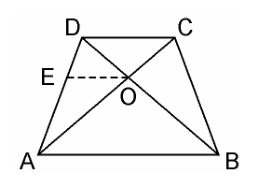Guru

# The diagonals of a quadrilateral ABCD intersect each other at the point O such that AO/BO = CO/DO. Show that ABCD is a trapezium. Q.10

• 0

The best way to solve the problem of Triangles of exercise 6.2 of class 10th math, i don’t know how to solve this problem please guide me to solve this problem The diagonals of a quadrilateral ABCD intersect each other at the point O such that AO/BO = CO/DO. Show that ABCD is a trapezium.

Share

1. Given, Quadrilateral ABCD where AC and BD intersects each other at O such that,

AO/BO = CO/DO.We have to prove here, ABCD is a trapezium

From the point O, draw a line EO touching AD at E, in such a way that,

EO || DC || AB

In ΔDAB, EO || AB

Therefore, By using Basic Proportionality Theorem

DE/EA = DO/OB ……………………(i)

Also, given,

AO/BO = CO/DO

⇒ AO/CO = BO/DO

⇒ CO/AO = DO/BO

⇒DO/OB = CO/AO …………………………..(ii)

From equation (i) and (ii), we get

DE/EA = CO/AO

Therefore, By using converse of Basic Proportionality Theorem,

EO || DC also EO || AB

⇒ AB || DC.

Hence, quadrilateral ABCD is a trapezium with AB || CD.

• 0